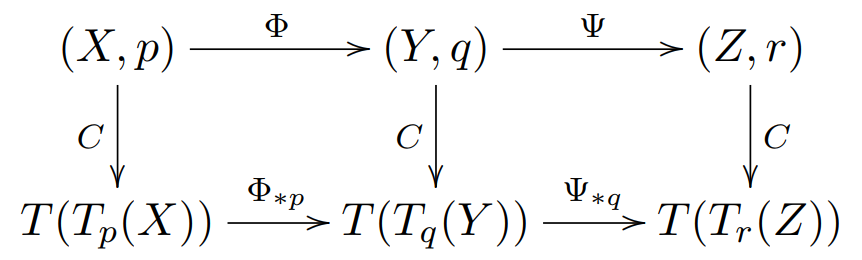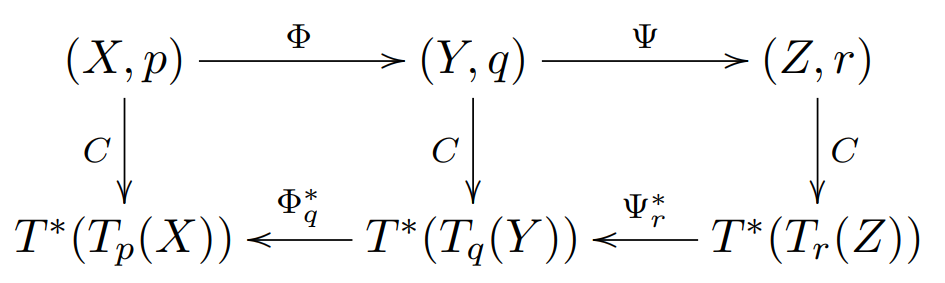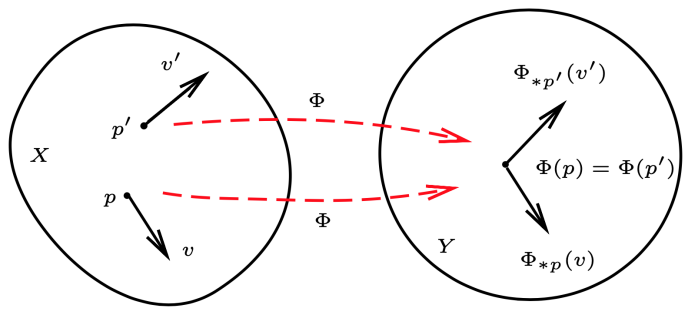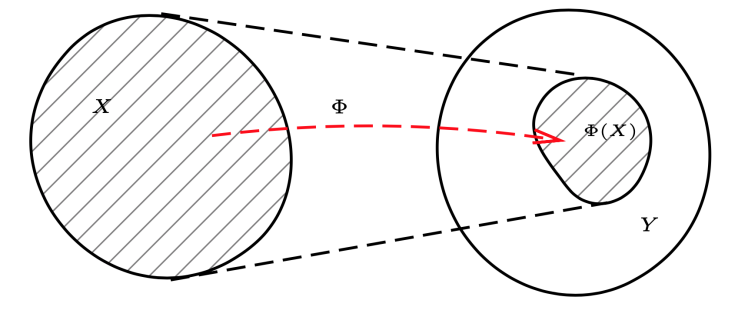## 张量场

### 一点处的张量

$\{e_i = \frac{\partial}{\partial x^i}|_p,i=1,\cdots,n\}\quad and\quad \{e^{*i} = dx^i|_p,i=1,\cdots,n\}$

$T^r_s(p)=\underbrace{T_pX\otimes\cdots\otimes T_pX}_{r}\otimes\underbrace{T^* _ pX\otimes\cdots\otimes T^* _ pX}_{s}$

$t_p = (t_p )^{i_1 \cdots i_r}_{\qquad j_1 \cdots j_s} e_{i_1} \otimes \cdots \otimes e_{i_r} \otimes e^{* j_1} \otimes \cdots \otimes e^{* j_s}$

#### 推前映射

$v \in T_p X$$p$ 点的推前映射是一个由 $p$ 点的切空间到 $q = \Phi(p)$点的切空间 $T_q Y$ 的映射：

$\Phi_{*p} : T_p X \rightarrow T_q Y , v \mapsto \Phi_{*p}(v)$

$\Phi_{*p} (v)(g) = v(\Phi^* g), \forall g \in\mathscr{F}(Y )$

$\forall g\in \mathscr{F}(Y),\ \forall p\in X\quad \Phi^*(g)(v) := g\circ\Phi(p)$

$\Phi_{*p}$$T_p X$ 上的一个线性映射。$\forall g\in \mathscr{F}(Y)$，那么 $\Phi^*g \in \mathscr{F}(X)$$v(\Phi^* g) \in \mathbb{R}$，来考虑 $\Phi_{*p}(v)$$Y$ 上的光滑函数 $g$ 映成实数，并且是线性映射，因此其应当是 $q = \Phi(p)\in Y$ 点的切矢量。即：$\Phi_{*p}(v) \in T_qY$

$\Phi_{*p}(x\otimes y) = \Phi_{*p}(x) \otimes \Phi_{*p}(y),\quad \forall x,y\in T(T_pX)$$q = \Phi(p),\quad r=\Psi\circ\Phi(p)$

$(\Psi\circ\Phi)_{*p} = \Psi_{*q}\circ \Phi_{*p}$

$(U,\varphi)$$X$ 上包含 $p$ 点的坐标卡，相应的坐标为 $\{x^i,i=1,\cdots,n\}$，自然基和其对偶基分别为：

$\frac{\partial}{\partial x^i}|_p\quad dx^i|_p\quad i=1,\cdots,n$

$(V,\psi)$$Y$ 上包含 $q$ 点的坐标卡，相应的坐标为 $\{y^I,I=1,\cdots,m\}$，自然基和其对偶基分别为：

$\frac{\partial}{\partial y^I}|_q\quad dy^I|_q\quad i=1,\cdots,m$

$\Phi_{*p}\frac{\partial}{\partial x^i}|_p$

\begin{aligned} \Phi_{*p}\frac{\partial}{\partial x^i}|_p(y^I) &= \frac{\partial}{\partial x^i}|_p (\Phi^*y^I)\\ &= \frac{\partial}{\partial x^i}|_p (y^I\circ\Phi)\\ &= \frac{\partial (y^I \circ \Phi \circ \varphi^{-1})}{\partial x^i}|_{\varphi(p)}\\ &= \frac{\partial (y^I \circ \psi^{-1}(\psi \circ \Phi \circ \varphi^{-1}))}{\partial x^i}|_{\varphi(p)}\\ \end{aligned}

$\Phi_{*p}\frac{\partial}{\partial x^i}|_p = \frac{\partial y^I}{\partial x^i}\frac{\partial}{\partial y^I}|_{q}$

$\frac{\partial y^I}{\partial x^i} = \frac{\partial (y^I \circ \psi^{-1}(\psi \circ \Phi \circ \varphi^{-1}))}{\partial x^i}|_{\varphi(p)}$

$t_p = t_p^{i_1\cdots i_r} \frac{\partial}{\partial x^{i_1}}|_p\otimes \cdots \otimes \frac{\partial}{\partial x^{i_r}}|_p$

$\Phi_{*p}t_p = t_p^{i_1\cdots i_r}\frac{\partial y^{I_1}}{\partial x^{i_1}}\cdots \frac{\partial y^{I_r}}{\partial x^{i_r}} \frac{\partial}{\partial y^{I_1}}|_p\otimes \cdots \otimes \frac{\partial}{\partial y^{I_r}}|_p$

#### 拖回映射

$\Phi^*_q (\omega) = \omega \circ \Phi_{*p} ,\ \forall \omega \in T^*_ q Y$$(\Psi\circ\Phi)_{r}^* = \Phi_q^*\circ \Psi_r^*$

$\Phi_q^*dy^I = \frac{\partial y^I}{\partial x^i}dx^i|_p$

### 张量场

$t = t^{i_1 \cdots i_r}_{\qquad j_ 1 \cdots j_ s}\frac{\partial}{\partial x^{i_1}} \otimes\cdots\otimes\frac{\partial}{\partial x^{i_r}}\otimes dx^{j_1} \otimes\cdots \otimes dx^{j_s}$

$t^{a_1\cdots a_r}_{\qquad b_1\cdots b_s}$

• $(0,0)$-型张量场就是流形上的 光滑函数，所有光滑函数的集合记为 $\mathscr{F}(X)$
• $(1,0)$-型光滑张量场称为 $X$ 上的 光滑切矢量场。所有光滑切矢量场的集合通常记为$\mathscr{X} (X)$
• $(0,1)$-型光滑张量场也称为 $1$-形式场。所有 $1$-形式场的集合通常记为 $\Lambda_1 (X)$
• 我们用 $\mathscr{T}^r_s(X)$ 表示流形 $X$ 上所有光滑 $(r,s)$-型张量场的集合。于是得到：

$\mathscr{F}(X) = \mathscr{T}_{0}^{0};\quad \mathscr{X}(X) = \mathscr{T}_{0}^{1};\quad \Lambda_1(X)=\mathscr{T}_{1}^{0}$

$t_p:\underbrace{T_p^*X\times\cdots\times T_p^*X}_{r}\times\underbrace{T_pX\times\cdots\times T_pX}_{s} \rightarrow \mathbb{R}$

$t:\underbrace{\Lambda_1(X)\times\cdots\times \Lambda_1(X)}_{r}\times\underbrace{\mathscr{X}(X)\times\cdots\times \mathscr{X}(X)}_{s} \rightarrow \mathscr{F}(X)$

$\mathscr{T}(X)=\bigoplus_{r,s=0}^\infty \mathscr{T}_s^r(X)$

#### 张量场的推前与拖回

• 非单射的情况
当映射 $\Phi$ $X\rightarrow Y$ 是多对一的情况，若 $X$ 上的矢量场在 $p$$p'$ 点的矢量被映为 $Y$$\Phi(p)$ 点的两个矢量。这样 $\Phi(p)$ 点被我们这个假定的推前映射指定了两个矢量，不能够在 $Y$ 上形成矢量场。• 非满射的情况
$\Phi$ 不是满射，至多给出 $\Phi(X)$ 上的矢量场。$\Phi^*: \mathscr{T}_s^0(Y) \rightarrow \mathscr{T}_s^0(X)$

$\Phi_* := (\Phi^{-1})^*$

### 切矢量场

$v_p : \mathscr{F}(X) \rightarrow \mathbb{R}$

$v : \mathscr{F}(X) \rightarrow \mathscr{F}(X),$

### 李括号

$u,v\in\mathscr{X}(X)$，它们也可以看作映射：$\mathscr{F}(X) \rightarrow \mathscr{F}(X)$。复合映射 $u\circ v$ 也是 $\mathscr{F}(X) \rightarrow \mathscr{F}(X)$ 的映射，但其并不满足 Leibniz 法则：

\begin{aligned} (u\circ v)(fg) &= u(v(fg)) = u(fv(g)+ gv(f))\\ &= f(u\circ v)(g) + f(u\circ v)(g) + u(g)v(f) u(f)v(g)\\ \end{aligned}

$[u,v] = u \circ v - v \circ u$

• 分配律

\begin{aligned} [\lambda_1u_1 + \lambda_2u_2,v] &= \lambda_1[u_1,v] + \lambda_2[u_2,v]\\ [u,\lambda_1v_1 + \lambda_2v_2] &= \lambda_1[u,v_1] + \lambda_2[u,v_2]\\ \end{aligned}

• 反对称律

$[u,v] = -[v,u]$

• 雅可比 (Jacobi) 恒等式

$[u,[v,w]] + [v,[w,u]] + [w,[u,v]] = 0$

$\mathscr{X} (X)$$\mathbb{R}$ 上的无穷维线性空间，故这个李代数是一个无穷维的李代数。这个无穷维的李代数所对应的群为 $\mathrm{Diff}(X)$，即光滑流形 $X$ 到其自身的所有微分同胚所形成的群。后面我们会有进一步的讨论。

$[u,v] = u^j\frac{\partial v^i}{\partial x^j}-v^j\frac{\partial u^i}{\partial x^j}\frac{\partial}{\partial x^i}$

$[u,v ]^i=u^j\frac{\partial v^i}{\partial x^j}-v^j\frac{\partial u^i}{\partial x^j}$

$[\frac{\partial}{\partial x^i},\frac{\partial}{\partial x^j}] = 0$